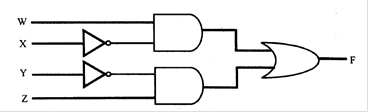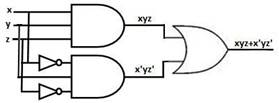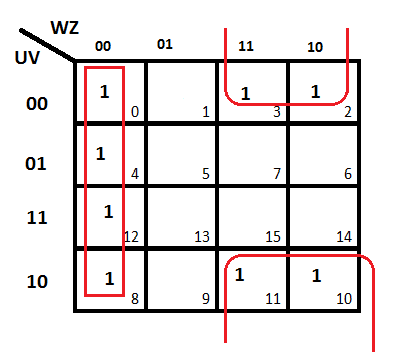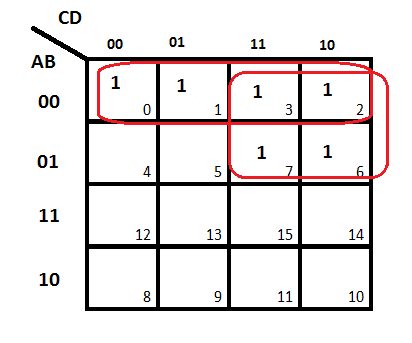### Boolean Algebra (OLD) - Test Papers

CBSE TEST PAPER-01

Class-12 Computer Science(Boolean algebra)

General Instruction: -

• Question No. 1 to 4 carry one marks,
• Question No. 5 to 8 carry two marks,
• Question No. 9 to 10 carry Three marks.

1. What do you mean by tautology and fallacy?
2. What is a logic gate? Name the three basic logic gates.
3. What is a truth table?
4. What is the significance of Principle of Duality?
5. State and Prove the idempotence law of Boolean algebra with the help of truth table.
6. State and prove Distributive Law using Boolean laws.
7. Draw logic circuit diagrams for the following :
i) xyz + x’yz’
8. Write the equivalent Boolean Expression for the following Logic Circuit.9. Reduce the following Boolean expression using K-Map
F(U,V,W,Z) = Σ(0,3,2,4,8,12)
10. Obtain the simplified form of a Boolean expression using K-Map.
F(A,B,C,D)=Σ(0,1,2,3,6,7)

CBSE TEST PAPER-01
Class-12 Computer Science(Boolean algebra)

1. If result of any logical statement or expression is always TRUE or 1, it is called Tautology and if the result is always FALSE or 0 it is called Fallacy.
2. A Gate is simply an electronic circuit which operates on one or more signals to produce an output signal. Three basic logic gates are as following Inverter (NOT Gate)
OR Gate AND Gate
3. Truth Table is a table which represents all the possible values of logical variables/statements along with all the possible results of the given combinations of values.
4. Principle of Duality is a very important principle used in Boolean algebra. This states that starting with a Boolean relation, another Boolean relation can be derived by :
Changing each OR sign (+) to an AND sign(.).
Changing each AND sign (.) to an OR sign(+).
Replacing each 0 by 1 and each 1 by 0
5. Idempotence law state that (a) X + X = X (b) X . X = X
(a) X + X = X
truth table:
 X X R 0 0 0 1 1 1
0 + 0 = 0 and 1 + 1 = 1 0.
From truth table it is prove that X + X = X
(b) X . X = X
truth table:
 X X R 0 0 0 1 1 1
0 + 0 = 0 and 1 + 1 = 1
From truth table it is prove that X . X = X
6. Distributive law state that (a) X(Y +Z) = XY + XZ (b) X + YZ = (X + Y)(X + Z)
a) X(Y +Z) = XY + XZ
LHS = X(Y+Z)
= XY+XZ
=RHS Hence the proof.
(b) X + YZ = (X + Y)(X + Z)
RHS= XX+XZ+XY+YZ
= X+XZ+XY+YZ
= X(1+Z)+XY+YZ
= X+XY+YZ
= X(1+Y)+YZ
= X+YZ = LHS Hence the proof.

7.8. WX’ +Y’Z

9.The reduced expression is W’Z’+WV’

10.The reduced expression is A’B’+A’C